# Countif function in Excel

COUNTIF function in excel counts cells meeting a single criterion. It will Count the Empty or the Blank Cells present in the Range. You can use COUNTIF Function to count or Calculate the number of Cells, the cells with dates, numbers, and text matching a specific criterion.

The COUNTIF function Supports the logical operators (>, <, <>, =) also with wildcards (*,? i.e the asterisk and the question mark). The Wildcards help in partial matching.

The Excel Countif function returns the number of cells within a supplied range, that satisfies the given criteria.

Excel Vlookup formula – Guidebook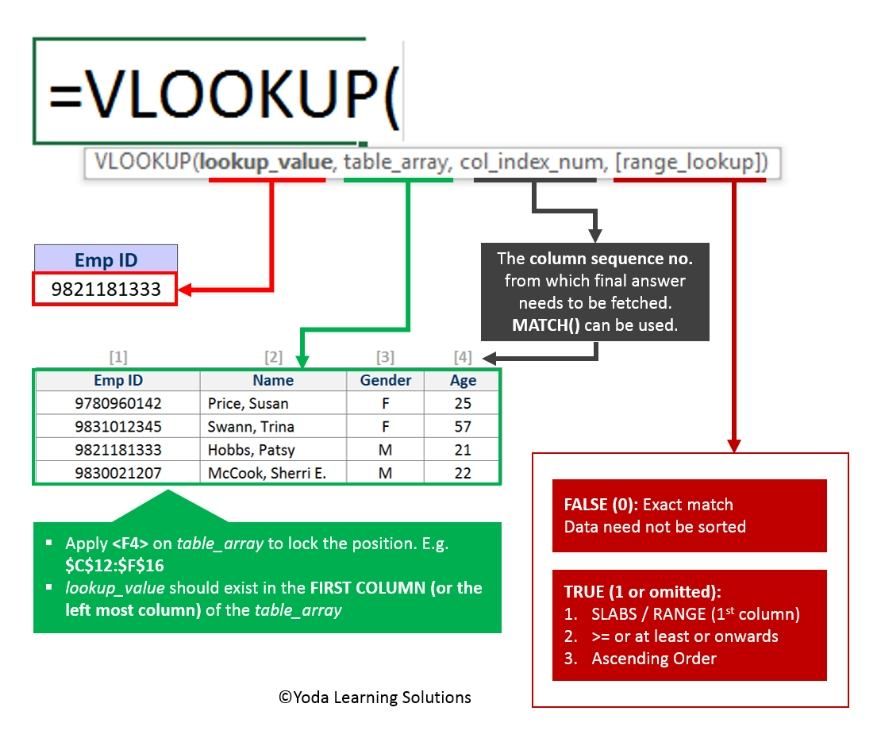If Yes, you will enjoy this guidebook on ‘Excel Vlookup Formulas’ – VLOOKUP, HLOOKUP, MATCH & INDEX.

## When to use Countif Function?

COUNTIF function can be used when you want to count the number of cells that meet specified criteria.

## What does it return?

It returns a value(number) that represents the number of cells that meet specified criteria in the selected range.

Purpose

• To Count the cells matching your criteria
• Return the value
• A number representing cells counted.

Syntax

COUNTIF(range, criteria)

• range – Defines one or several cells to count. You put the range in a formula like you usually do in Excel, e.g. A1:A20.
• criteria – Defines the condition that tells the function of which cells to count. It can be a number, text string, cell reference or expression. For instance, you can use the criteria like these: “10”, A2, “>=10”, “some text”.

Case Study: Let’s analyze the result grade sheet of students in which we want to find out how many students have got GPA 4. We can find this using Countif Function.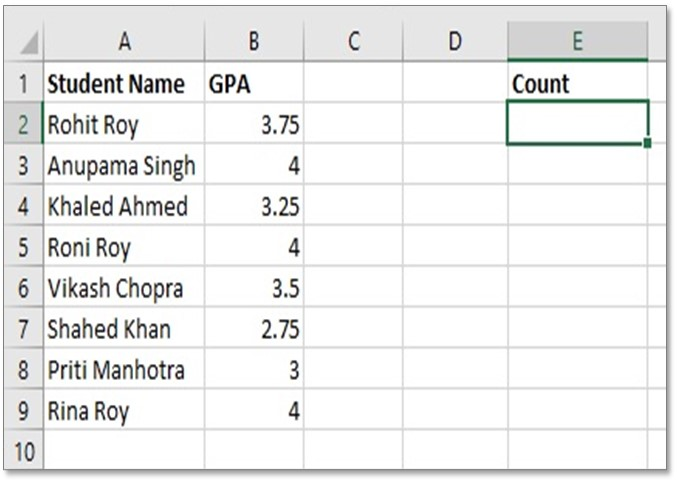## Steps on how to use Countif Function

Step 1: Create a table in Excel

Step 2: In Cell E2 we will calculate the number of students who have got GPA 4. type this formula in cell E2:

=COUNTIF(B2:B9,4)

15 Excel Data Cleaning Tricks Guidebook

Bored of downloading text heavy / copy-pasted eBooks? If Yes, you will enjoy this guidebook (43 pages)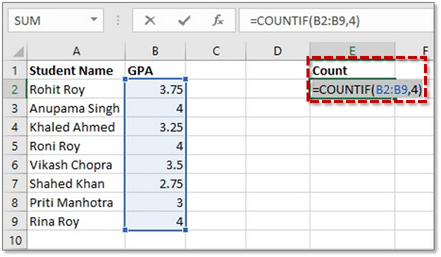Step 3: Now press ENTER, you will get the value 3 which is the number of students who got a GPA 4.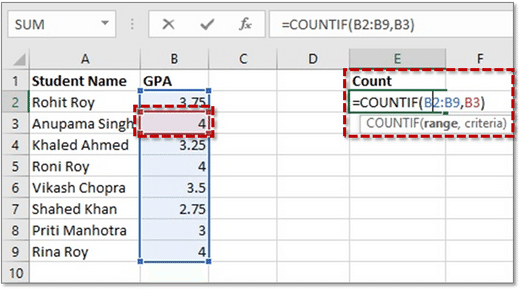Tip 1: In the Criteria portion of the formula, You can use also B3 to get the same result. Because cell B3 contains the value GPA 4. So, in that case, the formula would be:

=COUNTIF(B2:B9,B3)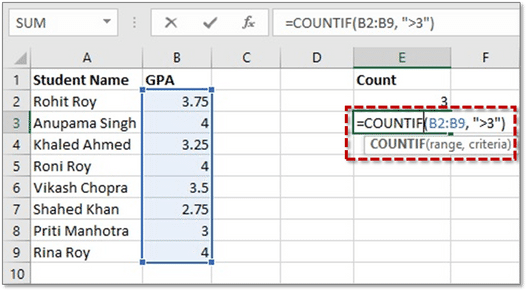Tip 2: Imagine that you want to find the number of students who got GPA of more than 3, then the formula would be:

=COUNTIF(B2:B9, “>3”)

So, we can also give mathematical conditions in criteria.

Tip 3: If you want to find those students whose name contains Roy, then use this formula and see the magic:

=COUNTIF(A2:A9, “*Roy”)

We use * before Roy which means find those values who have Roy at the end. By giving * means whatever exists before Roy it will count that.

Tip 4: We can also Sum 2 Countif results in another cell. Think you want to sum these 2 Countif results of =COUNTIF(B2:B9,B3) and COUNTIF(B2:B9, “>3”). To do so, you can use the formula given below:

=COUNTIF(B2:B9,B3)+COUNTIF(B2:B9, “>3”)

Excel Vlookup formula – GuidebookIf Yes, you will enjoy this guidebook on ‘Excel Vlookup Formulas’ – VLOOKUP, HLOOKUP, MATCH & INDEX.

## How to Use Excel COUNTIF Text

Supposing you have the same data as above, and you want to count the number of cells that contain the text ‘Roni Roy’.

Excel COUNTIF Text function will help to count the number of a specific text or parts of text cells.

1. Select a blank cell. Let’s say cell E2 (in our Case) and enter the formula

=COUNTIF(A2:A9,”*Roni Roy*”) into the Cell.

2. Now Press Enter
3. You’ll get the answer as 1

### COUNTIF greater than, less than or equal to

To count the no. of cells with values that are greater than or less than or equal to the specified number. Here, what you have to do is: Add a corresponding operator to the criteria that are shown in the table below.

Let’s check out an example of Excel Count if Greater than:

You can count the number of cells greater than or less than zero using this Formula:

In the given example shown above:

Steps for COUNTIF greater than, less than, or equal to:

• Select a Blank Cell, let’s say cell E2 (in our Case)
• Enter formula =COUNTIF (B2:B9,”>0″) into the Formula Bar
• Press Enter
• The Selected Blank cell will populate the number of Cells Greater than 0. In our case, we get the value as 8

Therefore, the Excel COUNTIF greater than 0 is value 8.

## Excel COUNTA function non Blank Cells

The Excel COUNTA function helps in returning the number of Cells that are non-Blank with the help of the Excel Counta Function. It helps in obtaining the Count of cells that contain text, empty text (“”), error values, logical values, and numbers. Excel Count if not blank does not count empty cells. The Excel COUNTBLANK function returns a count of empty cells in a specific range.

Steps for Excel COUNTA Function non-Blank Cells:

• Select a Blank Cell, let’s say cell E2 (in our Case)
• Enter formula =COUNTA(B2:B9) into the Formula Bar
• Press Enter
• The Selected Blank cell will populate the Excel Count of Cells if not blank. In our case, we get the value as 6

## Excel COUNTBLANK Function

The Excel COUNT BLANK will Count the Empty or the Blank Cells present in the Range. These Cells might contain errors, text and numbers etc. which are not considered. It only has the Formula which returns the empty text in Count.

If there are Blank Cells in your Dataset, you need to Count cells that are blank. If you need to count the cells that are Blank, you need to use the COUNTBLANK function.  You

The COUNTIF function in google sheets is a combination of IF function and the COUNT function in Google Sheets. With this Combination, you’ll be able to make a Count of numbers, text or values found in a selected range of Cells meeting a specified or a Single Criterion.

To get in-depth knowledge of other excel functions, check our Excel Dashboard Course. In this course training, we offer you online training videos with 24×7 online support.

15 Pivot Tables Tricks for Pros

Most Popular Tricks are #3, #7 & #12

### Alteryx Hotkeys - Alteryx Keyboard Shortcuts

Top 50+ Alteryx Shortcuts for Windows. Alteryx is popularly known as a Self-Service Analytics tool. Business users can build their data workflows…

### Step By Step Guide to Learn Alteryx

•